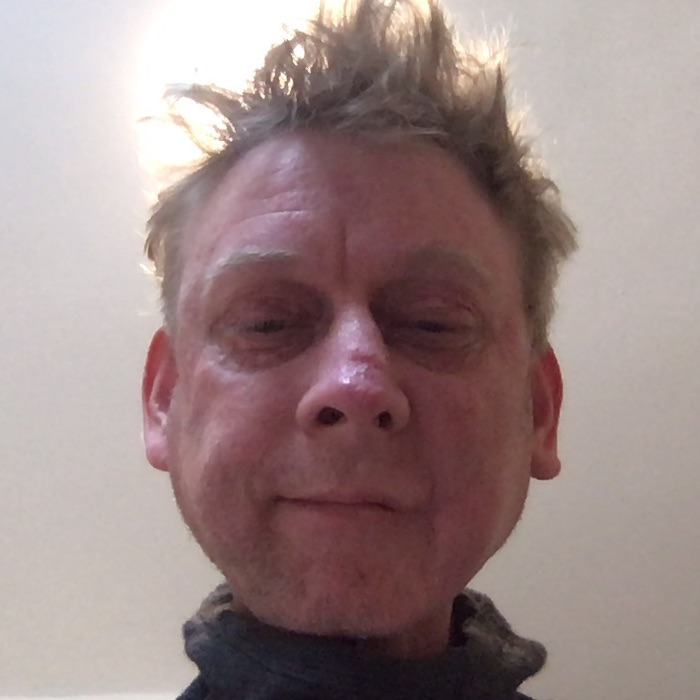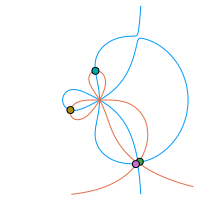# Sven-Erik EkströmI am a tenure track assistant professor (biträdande universitetslektor), since February 2021, at the Division of Scientific Computing, Department of Information Technology, Uppsala University, Uppsala, Sweden.

I defended my Ph.D. in scientific computing (numerical analysis) at Uppsala University in May 2018 with the thesis "Matrix-Less Methods for Computing Eigenvalues of Large Structured Matrices" (full text). Then, I was a postdoc at Athens University of Economics and Business and Bergische Universität Wuppertal, before receiveing the International postdoc grant (Spring Call 2019, 3.15 MSEK ≈ 340 kUSD) of the Swedish Research Council.

### Current plans:

My main research interest is spectral analysis of matrix sequences from PDE and FDE discretizations, and other structured matrices (especially Toeplitz-like), using matrix-less methods, the theory of GLT sequences, and other techniques. I especially like non-Hermitian matrix sequences with peculiar spectral behaviour. Some current projects are,• Parallel-in-Time (PiT): Spectral analysis of associated matrices;
• Fractional derivatives (FDE): Preconditioning for iterative solvers, and spectral analysis of discretization matrices;
• Asymptotic expansion (Matrix-less methods): Eigenvalues, eigenvectors, matrix-valued and multi-variate symbols, diagonal sampling matrices, perfect grids;
• Non-Hermitian symbols and matrix sequences: Spectral analysis of some special banded and non-banded Toeplitz and Toeplitz-like matrix sequences; e.g., $$\{T_n(f)\}_n$$ for symbols $$f(z)=-z+1+z^{-1}+z^{-2}+z^{-3}$$, $$z\in\mathbb{T}$$, $$f(z)=\alpha z^{-r}+\beta+\gamma z^s$$, $$r\neq s\in\mathbb{N}$$, $$\alpha,\beta,\gamma\in\mathbb{C}$$, or $$f(z)=z^{-1}(1-z)^\alpha$$, $$\alpha\in(1,2)$$.

My preferred research tools are Julia (with GenericLinearAlgebra.jl and GenericSchur.jl for high precision linear algebra and Pluto.jl for dynamic visualization and experiments), OEIS, RIES, Wolfram Alpha, a MacBook Pro 13", an AMD Ryzen Threadripper 3970X (32 Core, 256GB RAM, GeForce RTX 2060), clusters at UPPMAX, and an iPad Pro 12.9" with Notability.

In my spare time I enjoy packrafting, collecting tools, art, books, and artifacts, and restoring my house Lilla Säteriet (built 1819) located in Ekeby by.

Contact by email for questions or collaboration.